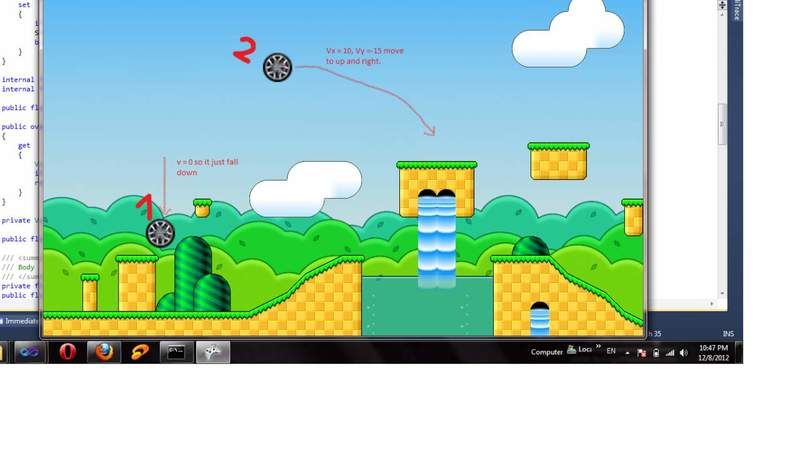# How to get angular velocity from force

1. There is a Rectangle (shape could change), and a force like F, that effect the rectangle at point P on its surface. i don't know how the shape will move after impulse, the center of mass is CM, the center of rotation is CR, the shape mass is m.
I'm really sorry to ask a question like this! but I'm a software developer and these are all i got. the problem is building a simulator for shape movement.

2. T = Iα, T = F × (P - CR) then α= F × (P - CR) / I
angular velocity = ?
Inertia = m * R^2 // the R is the shape radius or it depend to the CR? and is constant or not?
Linear Accelerate = F.(P - CR) / m // I'm not sure!
liner velocity is simply calculate if the above sentence was correct.
angular velocity and the angle from CR is unknown to me?

mfb
Mentor
Inertia = m * R^2
That is true for point-masses (and some special cases) only. For other shapes, you have to integrate or use specific formulas for those shapes.

Are you sure that you want a force, and not a transfer of momentum (force*time)?

The basic idea is to use conservation of momentum and angular momentum - in general, the object will begin to move and rotate at the same time. You can always write the movement as sum of both, where the rotation is around its center of mass (for a rectangle of uniform density, this is simply the geometric center). This gives three variables and three equations, so the system can be solved.

there is some time that the shape is connected to the other so it cannot rotate around its center of mass, in such this time i should use CR. about the Inertia the shape defined with some vectors. not an specified shape.
Yes that is going to be a momentum finally, but i didn't make any decision on it. because the game is real time and it will refresh every millisecond!

mfb
Mentor
If it is fixed, there is a single degree of freedom only (angular velocity), and you can use conservation of angular momentum around that center of rotation.

With finite timesteps, force*timestep has units of momentum :).

thanks again.
the shape is not fixed. when i hold the shape by mouse and move it side to side, the shape has a center of rotation as CR, am i right? when i release it, shape will rotate around its CM, but how to?
on that time calculation of the linear accelerate and Torque is not clear to me.
i even don't know how to use momentum to simulate the shape move!
until now by using only force i simulate gravity and so many kinematic formula. i have a circle that falls completely the same as what happen in the earth. but nothing else when its collide to the earth just stop moving.
please give me some formula, anything that help me to solve this problem, thanks.

I add this to my code: θ = ωt + 1/2 α t^2 and rotate the shape by time. but ω, Inertia even the Torque and linear accelerate are still missing!

and this :
ω = ω + α tthere is a sample. until now.

#### Attachments

mfb
Mentor
thanks again.
the shape is not fixed. when i hold the shape by mouse and move it side to side, the shape has a center of rotation as CR, am i right? when i release it, shape will rotate around its CM, but how to?
You have a forced rotation around some arbitrary point before?
You can calculate the total momentum (just use the velocity of the CM point) and angular momentum (you can use the angular velocity around CR) of the object at the time of release, and use those to calculate propagation afterwards.

i have a circle that falls completely the same as what happen in the earth. but nothing else when its collide to the earth just stop moving.
If the collision is elastic, it will invert its vertical velocity (or the component vertical to the surface, if the surface is not horizontal).